Bayesian Inference for Sparse VAR(1) Models, with Application to Time Course Microarray Data | OMICS International
Journal of Biometrics & Biostatistics

Like us on:

All submissions of the EM system will be redirected to Online Manuscript Submission System. Authors are requested to submit articles directly to Online Manuscript Submission System of respective journal.

# Bayesian Inference for Sparse VAR(1) Models, with Application to Time Course Microarray Data

Guiyuan Lei1,2, Richard J Boys1,2*, Colin S Gillespie1,2, Amanda Greenall2,3 and Darren J Wilkinson1,2

1School of Mathematics & Statistics, Newcastle University, Newcastle upon Tyne, NE1 7RU, UK.

2Centre for Integrated Systems Biology of Ageing and Nutrition (CISBAN), Newcastle University

3Institute for Ageing and Health, Newcastle University, Campus for Ageing and Vitality, Newcastle upon Tyne, NE4 5PL, UK

*Corresponding Author:
Richard J. Boys
School of Mathematics & Statistics
Newcastle University
Newcastle upon Tyne, NE1 7RU, UK
E-mail: [email protected]

Received Date: October 22, 2011; Accepted Date: November 21, 2011; Published Date: December 25, 2011

Citation: Lei G, Boys RJ, Gillespie CS, Greenall A, Wilkinson DJ (2011) Bayesian Inference for Sparse VAR(1) Models, with Application to Time Course Microarray Data. J Biomet Biostat 2:127. doi: 10.4172/2155-6180.1000127

Copyright: © 2011 Lei G, et al. This is an open-access article distributed under the terms of the Creative Commons Attribution License, which permits unrestricted use, distribution, and reproduction in any medium, provided the original author and source are credited.

Visit for more related articles at Journal of Biometrics & Biostatistics

#### Abstract

This paper considers the problem of undertaking fully Bayesian inference for both the parameters and structure of a vector autoregressive model on the basis of time course data in the ``p>> n scenarioâ€™â€™. The autoregressive matrix is assumed to be sparse, but of unknown structure. The resulting algorithm for dynamic Bayesian network inference is shown to be highly effective, and is applied to the problem of dynamic network inference from time course microarray data using a dataset concerned with the transient response of budding yeast to telomere damage.

#### Keywords

Var(1); MCMC; Microarray

#### Introduction

This paper considers the problem of carrying out fully Bayesian inference for both the parameters and structure of a vector autoregressive model on the basis of time course data. Although in no way essential to the analysis, the techniques are particularly well suited to time series of very high dimension, and are shown to work well even in the scenario where the number of observations is small relative to the dimension of each observation. The autoregressive matrix is assumed to be sparse, but of unknown structure. The non-zero structure of the matrix can be associated with a directed graph representing a dynamic network of interactions. The interpretation of the inferred network is closely related to the concept of Granger causality . A reversible jump MCMC algorithm is developed in order to carry out simultaneous inference for the parameters and structure of the model in a fully Bayesian manner. This dynamic Bayesian network inference algorithm is shown to be highly effective, and is applied to the problem of dynamic network inference from time course microarray data using a dataset concerned with the transient response of budding yeast to telomere damage.

There is a large literature on network inference from microarray data [2,3]. However, most of this literature is concerned with static network inference using non-dynamic data, where the inference of causal effects is distinctly problematic. Bayesian methods for complex bioinformatics applications are reviewed in . Although the essential problem of dynamic Bayesian network inference using time course microarray data has been considered by several authors , most of the literature is concerned with the fitting of discrete variable networks to discretised data. The fitting of a sparse VAR(1) model was considered by , but there a shrinkage estimation technique was used to obtain a simple point estimate of the network structure. A Bayesian approach to estimating the structure of an autoregressive model is considered in , but the emphasis there is very different, and is very much focussed on the scenario where the dimension of the problem is small relative to the number of observations. This paper therefore represents the first fully Bayesian approach to dynamic network inference from time course microarray data using (normalised) continuous measurements.

In the next section, we describe the vector autoregressive model and its Bayesian analysis via a reversible jump algorithm. The performance of the algorithm is assessed via a simulation study in Section 3.1. There follows an application to a time course microarray dataset concerned with the transient response of budding yeast to telomere damage and some conclusions.

#### Materials and Methods

We describe the (discrete) time evolution of the k-dimensional process yt = (yt,1,..,yt,2,.., yt,k)T by using a first order vector autoregressive model

yt= µ +A(yt-1-m) + et , t = 2,3,…,T                                              (1)

where µ is the k-dimensional (time invariant) mean vector, A is the k×k matrix of autoregressive coefficients and the et are error terms. We will consider models in which the A=(aij) matrix is sparse, that is, in which relatively few of the k components of the process interact over time. From the perspective of dynamic graphical modelling, the nonzero elements aij can be interpreted as edges from variable Yj to variable Yi, and zero elements as the absence of edges. The main inferential task considered here for the analysis of VAR(1) models is to make appropriate statements about the sparsity structure of the A matrix.

We assume for the moment that the errors et are independent and normally distributed and that their components are independent with precision τ, that is, et ∼Nk(0,τ-1Ik). Suppose that the data consist of R independent realisations of the process and denote this by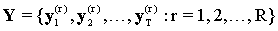. The Markovian structure of the model gives the likelihood function as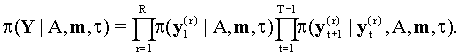A marginal model for the initial observations y1 could be specified in several ways. For example, if a prior distribution π (y0) were specified for the process before observation begins, then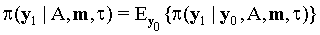. However, we seek a likelihood function which retains the simple structure given by the autoregressive model. Taking a marginal model which is uniform achieves this result. Other large sample arguments (T large) suggest that little information will be lost if the contribution of this marginal model is ignored, with this essentially conditioning the likelihood on the observed value of y1. In this paper, we will simplify the likelihood function by ignoring the contribution of this marginal model.

Prior distribution

We assume a priori independence between the parameters in the model, giving prior distribution

π(A,μ,τ) = π(A)π(μ)π(τ), and where possible, the (marginal) prior distributions are chosen to yield semi-conjugate updates.

The prior distribution for the autoregressive matrix A = (aij) is formed by assuming independence across its elements. Further, we assume that an edge is present from variable Yj to variable Yi (i.e aij ≠ 0) with probability p and that these non-zero elements follow a normal distribution with mean c and standard deviation d. We also take a normal N(m,q-1) prior distribution for the process mean µ which allows a priori correlation between variables and a gamma Γ(g,h) prior distribution for the precision of the error terms.

This prior distribution places a binomial B(k2,p) distribution on the number of edges in the network and, as the network is believed to be sparse, we take p=1/k. In the subsequent analyses, we also take the prior for the non-zero elements of A to have mean c = 0 and standard deviation d = 0.2. We input weak prior information about the process mean by taking µ = 0 and q = 0.01Ik and for the error terms, by taking g = 1 and h = 0.01.

Posterior distribution

The complexity of the model requires that we adopt a Monte Carlo Markov chain (MCMC) strategy to obtaining the posterior distribution for the model parameters. A complicating feature is that variable dimension moves are required to add or delete edges within the A matrix. These moves have the effect of replacing a zero element aij with a non-zero value and vice versa. Although the MCMC scheme could be initialised by simulating a realisation of the autoregressive matrix A from its prior distribution, given the ''known'' sparsity of the network, we have chosen to initialise the scheme by taking A as the null matrix.

Outline of MCMC scheme

At each iteration of the MCMC algorithm, a fixed scan is performed of the following moves: [(a)]

1. update the autoregressive matrix A by proposing to add or delete an edge;

2. update all non-zero elements of A in turn;

3. update the process mean vector m by using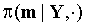;

4. update the error precision τ by using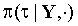.

Move (a): In Move (a), an element of A is selected at random, say aij where i and j are independent discrete uniform numbers from {1,2,..,k}. If this element is zero then an edge is proposed from variable Yj to variable Yi and a new value u proposed for aij from its full conditional distribution in the larger network, namely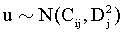. Following the arguments in , this reversible jump move is accepted with probability min(Aa,1),

where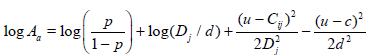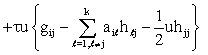(2)

and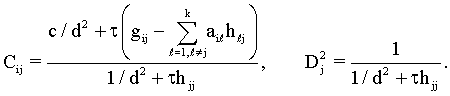(3)

Note that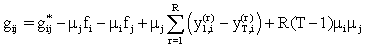(4)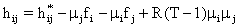(5)

depend on datasummaries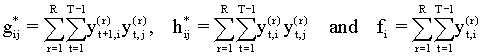(6)

which can be pre-calculated prior to starting the main loop of the MCMC algorithm.

If the selected element aij is non-zero then a deletion of this edge is proposed and accepted with probability min(Ad,1), where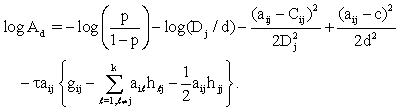(7)

If this move is accepted, we set aij = 0 .

Moves (b)-(d): These moves consist of Gibbs steps with

• Move (b):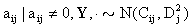• Move (c):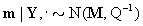, where

Q = q+R(T-1)τ(Ik-A)T(Ik-A),                                                                                                     (8)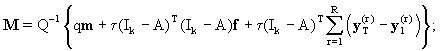(9)

• Move (d):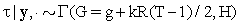, where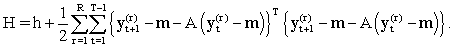(10)

In this paper we consider a vector autoregressive model which assumes independent errors and a common error variance. This model is particularly suited to our intended application of microarray data analysis and other problems in the ''p >> n paradigm'' due to its sparse parsimonious form. The sparsity and parsimony can be exploited in the implementation of the inference algorithm, and this can make orders of magnitude difference to computational speed and efficiency. However the model (and MCMC scheme) can be generalised to allow correlations in the error structure in a straightforward manner. The details for a generalisation of the model in which the error structure is modelled by a Wishart distribution are given in the Web Appendix.

#### Results

Simulation study

A simulation study was used to assess the ability of the MCMC algorithm to correctly detect edges within networks and also its accuracy in inferring the size of the non-zero elements of the autoregressive matrix. The algorithms were also assessed for their convergence properties by using formal and graphical summaries. Studying the trace of the log-likelihood function and the number of edges in the network was found to be particularly useful in diagnosing convergence. Various scenarios were considered and we report here typical results for a process with a small number of variables (k =5) and another with a fairly large number of variables (k =100).

Process with k =5 variables: We consider the 5-dimensional process shown in (Figure 1), with autoregressive matrix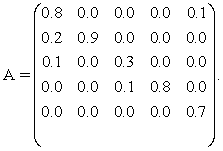(11)

Note that the eigenvalues of A lie between -1 and +1 and so the process has a stationary distribution. Also the matrix is sparse and, although it has some large elements, it also has some small (nonzero) elements which might prove to be difficult to detect by using the algorithm.

We begin by considering inference based on a dataset Y of R = 2 time series, each with T = 500 observations. The data were simulated assuming a mean µ = (2=,2,2,2,2)T and an error precision τ = 4. The MCMC algorithm was run for 100K iterations and typically required only a short burn-in of 200 iterations and then a thinning of 10 to obtain (almost uncorrelated) values from the posterior distribution. This takes 36 CPU seconds on a 3.4GHz PC (using C code available from the authors on request). Figure 2 shows some MCMC diagnostics for a typical run post burn-in. It gives the trace plot, autocorrelation plot and histogram (or denisty) for the dimension-free variables: the number of edges in the network and the log-likelihood function.

We now compare this posterior distribution with the results of a typical run of the algorithm. Firstly, we look at the process mean m. This had posterior mean (2.10,2.35,1.99,2.08,1.97)T with componentwise posterior standard deviations (008,0.19,0.03,0.08,0.5). Thus this parameter is quite accurately estimated from these data. The same is also true for the error precision parameter τ, with posterior mean (sd) of 3.97 (0.08).

Now we examine whether the posterior distribution correctly summarised the network between variables. The posterior output gave the matrix of probabilities for edge presence as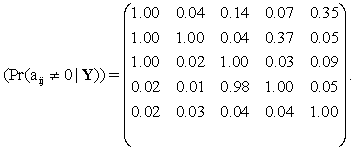(12)

Of the 9 edges in the true network (Figure 1), this matrix has 8 of these edges with presence probability exceeding 0.98 and remaining edge (from Y5 to Y1) with a fairly low presence probability. Note that this latter edge corresponds to one of the elements in A with a low signal (a15 = 0.1). All edges which are missing from the true network have a very low posterior presence probability.

Turning to the elements of the autoregressive matrix, conditional on each relevant edge being present, the posterior means of the elements of A were (to two decimal places)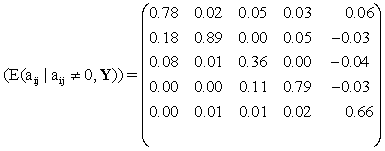(13)

with posterior standard deviations for each element being no more than 0.03. Thus the posterior distribution produces reasonably accurate estimates for the true autoregressive matrix.

The simulation study was repeated to assess the algorithm’s inferential accuracy in increasingly data-poor scenarios. Specifically, R=2 time series were simulated from the network using the same value of (A,µ,τ), first with T=100 observations, then with T=25 observations and subsequently with T=15 and with T=5 observations.

We assess the resulting posterior distribution for the edges in the network by calculating the number of edges whose presence probability exceeds a particular threshold; see (Table 1). Also of interest is the proportion of true edges identified in this way. Here, for each threshold value, the output from each scenario only identified edges that were in the true network. Thus each scenario-threshold combination produced a 100%-value for the predictive value positive. Further inspection of the MCMC output showed that all four strong signals (with aij > 0.3) were correctly detected when using time series of length at least T=25 observations, with only one of these signals being missed when using the T=15 series.

 T (l)2-6 Threshold 500 100 25 15 5 0.9 8 6 5 2 0 0.5 8 7 5 5 1

Table 1: For the 5 variable network: the number of (true) edges whose posterior presence probability exceeds the stated threshold when using R = 2 times series with various numbers of observations T.

The results for the shortest series (T = 5) show how difficult it can be to detect these signals with such little information: with a 0.9 threshold, no true edges were detected and with a 0.5 threshold, only one true edge was detected. Clearly, if only short time series are available then accurate inferences can only be obtained by increasing the number of replicates. For example, in a simulation study with T = 5, increasing the number of replicated time series to R = 6 resulted in the reliable detection of strong signals using a 0.95-threshold but the weaker signals were still missed. However, all (and only) correct edges were detected by using a 0.5-threshold.

Process with k=100 variables

We now consider a much larger network containing 100 variables and take the autoregressive matrix to be sparse with non-zero elements above and below the diagonal: ai,i+1 = ai,i+1 = 0.4, i = 1,2,…,99. This network has a total of 198 edges. We retain the same structure for the mean vector and error precision, namely µ = (2,2,..,2)T and τ = 4 and begin by analysing simulated data from R = 2 times series, each with T = 500 observations.

Not surprisingly the MCMC scheme for this much larger network required many more iterations to achieve convergence and exhibited much stronger autocorrelation. In a typical run of 1M iterations, after a burn-in of 50K iterations, the chain still showed fairly strong autocorrelations after thinning by every 100 iterates. Convergence was also confirmed by the very strong overlap of common edges in the inferred networks (using a presence probability threshold of 0.3) obtained from several independent runs of the algorithm.

Using a posterior presence probability threshold of 0.8, the MCMC output detected 198 edges in inferred network of which only one is a false positive. Thus only one edge in the true network was not detected. Also, the posterior distributions for the mean m and error precision τ were located around the true values. Given the size of the network, the matrix of presence probability for edges and the posterior mean of A (conditioned on relevant edges being present) are best viewed as graphs; see (Figure 3).

Figure 3: Plots for the 100 variable network. Top: posterior probability of edge presence (Pr(aij ≠ 0| Y)). Bottom: posterior mean for the autoregressive parameters given edge presence (E(aij | aij≠ 0,Y)).

Both graphs clearly show structure just above and below the diagonal and confirm that the algorithm has largely recovered the true network for the autoregressive matrix A. More detailed summaries of the accuracy of the inferred network are given for this R = 2 , T = 500 scenario in (Table 2). It shows that the predictive value positive is very high (above 95%) for thresholds of 0.3 and above and that the autoregressive parameters for the true edges are recovered to a reasonable accuracy -- all within 0.1 and 91.9% within 0.05. For all thresholds given in the Table, the inferred network contained the true network as a subgraph though, as expected, lower thresholds produced networks with more false positives.

 Probability threshold Scenario 0.1 0.3 0.5 0.7 R = 2 No. of edges detected 285 208 202 199 T = 500 No. of true edges detected 198 198 198 198 Predictive value positive 69.5% 95.2% 98.0% 99.5% No. of true edges with | E (aij | aij ¹ 0,Y) - 0.4 | < 0.1) 198 198 198 198 | E (aij | aij ¹ 0,Y) - 0.4 | < 0.05) 182 182 182 182 R = 10 No. of edges detected 254 208 199 194 T = 15 No. of true edges detected 198 198 197 194 Predictive value positive 78.0% 95.2% 99.0% 100% No. of true edges with | E (aij | aij ¹ 0,Y) - 0.4 | < 0.1) 171 171 171 171 | E (aij | aij ¹ 0,Y) - 0.4 | < 0.05) 115 115 115 115 R = 6 No. of edges detected 52 16 8 2 T = 5 No. of true edges detected 38 14 7 2 Predictive value positive 73.1% 87.5% 87.5% 100% No. of true edges with | E (aij | aij ¹ 0,Y) - 0.4 | < 0.1) 38 14 7 2 | E (aij | aij ¹ 0,Y) - 0.4 | < 0.05) 22 10 7 0

Table 2: For the 100 variable network: the number of edges detected using different presence probability thresholds, number of these edges which are in the true network, the positive predictive value (their ratio) and number of edges in the true network whose posterior mean for aij is within a given tolerance of the correct value.

We also investigated the performance of the algorithm for the other (R,T) combinations considered for the small 5-variable network. The performance of the MCMC schemes were similar to that for the 100-variable network described earlier. Again convergence was confirmed by the very strong overlap of inferred networks obtained from several independent runs of the algorithm. Summaries of the performance of the algorithm in these scenarios is given in (Table 2) and graphical summaries in (Figure 3).

For the (R = 10,T = 15) dataset, the algorithm reliably detected the edges in the true network, with a predictive value positive of over 95% when using presence probability thresholds of 0.3 and above. Also, for all the thresholds considered in (Table 2), nearly all (171/198=86%) of the autoregressive coefficients in the true network had mean values within 0.1 of their true value and 115/198 = 58% within 0.05. Figure 3 confirms the excellent true positive detection rate, and also shows that that few false edges would be detected when using thresholds of 0.3 and above. However, it also displays a noisy random pattern of higher values of the aij. However, taken together these Figures show that the analysis does reveal the correct network structure and accurate values for the autoregressive coefficients for the most plausible network structure.

The analysis of data from the most data-poor scenario (R = 6,T = 5) showed that it did not have sufficient information to capture the full network structure nor the autoregressive parameters; see (Figure 3). For example, for thresholds of 0.3 and above, the edges detected were largely correct (with predicted true positives exceeding 87%) and even for the 0.1 threshold, 73% of the edges detected were in the true network. However overall only a small number of the true edges were detected. As before, the autoregressive coefficients for those edges detected by the analysis were fairly accurately determined.

Conclusions from the simulation study: The analysis for both small and large networks showed that the algorithm performs well except in extremely data-poor scenarios. In general, using a presence probability threshold of 0.3 detected a high proportion of edges in the true network and relatively few false edges, with predicted true positive rate of around 90% or greater. Also, with smaller datasets, only a relatively small proportion of true edges were detected. Of course, judgements of whether a dataset is data-rich or data-poor depend not only on the number of times series and their length but also to the size of the variability in the error process. Here we have considered the errors to have a precision around τ = 4 . If this precision were much higher then nominally data-poor scenarios would in fact yield quite accurate inferences for the network structure and the associated autoregressive matrix. Clearly, the reverse will happen for error processes with low precision.

The results for small T are of practical interest as, in the intended application to time course microarray data, the high cost of arrays precludes very high sampling frequencies or large numbers of replicates. Values of T between 5 and 30 are typical, as are values of R between 1 and 5. Consequently, in practice, data-poor scenarios are the norm. However, these results indicate that even in such circumstances, it is reasonable to expect to uncover a good proportion of the strongest interactions using currently available data. Furthermore, the cost of microarray technology is falling rapidly and is likely to lead to much larger datasets which, in turn, will result in our technquies uncovering much weaker interactions within networks.

Analysis of a yeast genetic network

Having established the utility of our modelling approach and MCMC algorithm in the context of simulated data, we now turn our attention to the analysis of a real microarray dataset of practical interest. The data relates to an experiment designed to investigate the response of the budding yeast Saccharomyces cerevisiae to a certain kind of DNA damage caused by ''telomere-uncapping''. The ultimate goal of the experimental programme is to gain better understanding of the eukaryotic response to telomere damage. The experiment uses a temperature sensitive mutant yeast strain known as cdc13-1. This strain has a defect in the essential CDC13 gene which causes Cdc13 (a telomere-capping protein) to become defective above a threshold temperature. By raising the temperature above this threshold at the start of the experiment, the cellular response to telomere-uncapping can be observed. The data consists of 6 time series, each consisting of 5 time points. Each of the 30 measurements is a single yeast Affymetrix gene expression microarray, giving the mRNA levels of around 6,000 S. cerevisiae genes. The experiment and the preliminary analysis of the data are described in detail in .

By construction, the experiment is designed to stimulate the dynamic response of those genes involved in the telomere uncapping response, and so only a small proportion of the 6,000 genes present on the array are expected to exhibit interesting dynamics that will allow inference of network connectivity. Our model has been fitted to the 150 genes most significantly differentially expressed (and therefore exhibiting the most interesting dynamic behaviour) together with 33 other genes of particular interest to the experimental lab. The preprocessing of the microarray data and the identification of the differentially expressed genes was carried out using Bioconductor (www.bioconductor.org), and is described briefly in , and in more detail in .

Ten independent MCMC runs were made with 1M iterations to burn-in and then a further 1M iterations. After thinning by taking every 1K iterate, the chains still suffered from moderately high autocorrelation; see (Figure 4) for MCMC diagnostics of the number of edges in the network and the log-likelihood function for one of the chains post burn-in. As before, convergence was also confirmed by the very strong overlap of common edges in the inferred networks (using a presence probability threshold of 0.3) obtained from these independent runs of the algorithm. Pooling these thinned chains gives 10K realisations from the posterior distribution on which to base our analysis. Graphs of the posterior probability of edges presence and the (conditional) mean value of autoregressive matrix A are given in (Figure 5).

Figure 5: Plots S. Cerevisiae gene-gene interaction network of 183 genes. Top: posterior probability of edge presence (Pr(aij ≠ 0|Y)). Bottom: posterior mean for the autoregressive parameters given edge presence (E(aij|aij≠ 0,Y)).

We visualize our inferred networks using Cytoscape (www.cytoscape.org), an open source bioinformatics software platform for visualizing molecular interaction networks and biological pathways. The cytoscape (binary) file for the network produced when including edges whose presence probability is higher than 0.1 is provided in the Web Appendix. The smaller network based on presence probabilities greater than 0.3 is shown in (Figure 6). In this network, COS12 appeared as a gene which was highly connected thus potentially implicating it in the downstream regulation of many other genes in the time course. The COS12 gene is located sub-telomerically and encodes a protein of unknown function. However, it is a member of a family of genes with conserved sequence and has orthologues in other fungal species, and hence it is likely to be important. It will therefore be of interest to carry out further experimentation to determine whether Cos12 does indeed possess a previously unknown regulatory role in the response to telomere uncapping.

Figure 6: Inferred network for the S. Cerevisiae gene-gene interaction data when using an edge presence probability threshold of 0.3.

#### Conclusions

This paper has shown that it is possible to simultaneously estimate the parameters and structure of a VAR(1) model, using relatively short high-dimensional time series such as time course microarray data. The sparsity structure of these models has a natural interpretation as a dynamic network of gene--gene interactions. The information provided by the fully Bayesian analysis is very rich, including marginal posterior probabilities of edge inclusion. The methods were shown to perform well on simulated data, and then applied to an interesting time course microarray dataset. The analysis of the real data resulted in an inferred interaction network with interesting structure that is now the focus of follow-up experimental work.

#### Acknowledgements

We wish to thank Dan Swan (Newcastle University Bioinformatics Support Unit) and David Lydall for helpful discussions. The authors are affiliated with the Centre for Integrated Systems Biology of Ageing and Nutrition (CISBAN) at Newcastle University, which is supported jointly by the Biotechnology and Biological Sciences Research Council (BBSRC) and the Engineering and Physical Sciences Research Council (EPSRC).

#### References

Select your language of interest to view the total content in your interested language

### Article Usage

• Total views: 12287
• [From(publication date):
December-2011 - Nov 19, 2019]
• Breakdown by view type
• HTML page views : 8434Can't read the image? click here to refresh